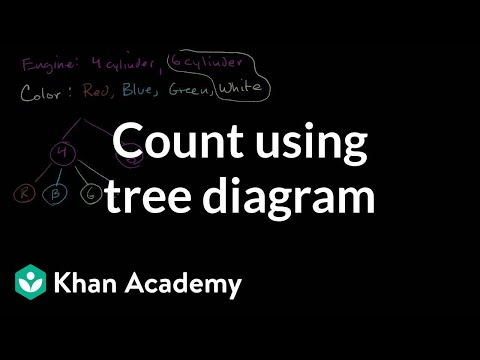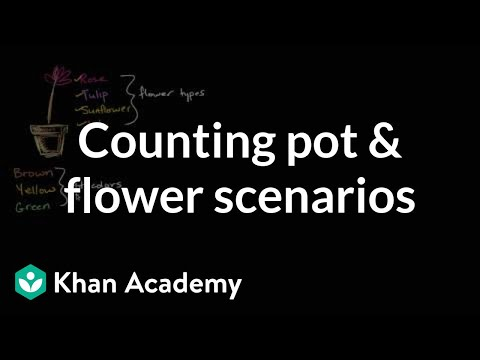Video

# Sample spaces for compound events (Full video)

Description: Explore the notion of a "sample space." See a sample space represented as a tree diagram, table, and list. If you're doing a trial, something that is probabilistic, a trial or an experiment, a sample space is just the set of the possible outcomes. So if you're talking about a coin flip, well then the sample space is going to be the set of all the possible outcomes. Or you could even construct, once you know all of the possible outcomes even if they aren't equally likely, you could say well let's create a probability distribution.

### Other videos you might be interested in### Count outcomes using tree diagram (Full video)